# Improvement of Pandas data processing performance using Multi-threading with the Queue (Another crossover of Space Stone, Reality Stone & Power Stone)

Today, we’ll discuss how to improve your panda’s data processing power using Multi-threading. Note that, we are not going to use any third party python package. Also, we’ll be using a couple of python scripts, which we’ve already discussed in our previous posts. Hence, this time, I won’t post them here.

Please refer the following scripts –

```a. callClient.py
b. callRunServer.py
c. clsConfigServer.py
d. clsEnDec.py
f. clsL.py
g. clsParam.py
h. clsSerial.py
i. clsWeb.py```

Please find the above scripts described here with details.

So, today, we’ll be looking into how the multi-threading really helps the application to gain some performance over others.

Let’s go through our existing old sample files –And, we’ve four columns that are applicable for encryption. This file contains 10K records. That means the application will make 40K calls to the server for a different kind of encryption for each column.

Now, if you are going with the serial approach, which I’ve already discussed here, will take significant time for data processing. However, if we could club a few rows as one block & in this way we can create multiple blocks out of our data csv like this –As you can see that blocks are marked with a different color. So, now if you send each block of data in parallel & send the data for encryption. Ideally, you will be able to process data much faster than the usual serial process. And, this what we would be looking for with the help of python’s multi-threading & queue. Without the queue, this program won’t be possible as the queue maintains the data & process integrity.

One more thing we would like to explain here. Whenever this application is sending the block of data. It will be posting that packed into a (key, value) dictionary randomly. Key will be the thread name. The reason, we’re not expecting data after process might arrive in some random order wrapped with the dictionary as well. Once the application received all the dictionary with dataframe with encrypted/decrypted data, the data will be rearranged based on the key & then joined back with the rest of the data.

Let’s see one sample way of sending & receiving random thread –The left-hand side, the application is splitting the recordset into small chunks of a group. Once, those group created, using python multi-threading the application is now pushing them into the queue for the producer to produce the encrypted/decrypted value. Similar way, after processing the application will push the final product into the queue for consuming the final output.

This is the pictorial representation of dictionary ordering based on the key-value & then the application will extract the entire data to form the target csv file.Let’s explore the script –

1. clsParallel.py (This script will consume the split csv files & send the data blocks in the form of the dictionary using multi-threading to the API for encryption in parallel. Hence, the name comes into the picture.)

 ``` 1 2 3 4 5 6 7 8 9 10 11 12 13 14 15 16 17 18 19 20 21 22 23 24 25 26 27 28 29 30 31 32 33 34 35 36 37 38 39 40 41 42 43 44 45 46 47 48 49 50 51 52 53 54 55 56 57 58 59 60 61 62 63 64 65 66 67 68 69 70 71 72 73 74 75 76 77 78 79 80 81 82 83 84 85 86 87 88 89 90 91 92 93 94 95 96 97 98 99 100 101 102 103 104 105 106 107 108 109 110 111 112 113 114 115 116 117 118 119 120 121 122 123 124 125 126 127 128 129 130 131 132 133 134 135 136 137 138 139 140 141 142 143 144 145 146 147 148 149 150 151 152 153 154 155 156 157 158 159 160 161 162 163 164 165 166 167 168 169 170 171 172 173 174 175 176 177 178 179 180 181 182 183 184 185 186 187 188 189 190 191 192 193 194 195 196 197 198 199 200 201 202 203 204 205 206 207 208 209 210 211 212 213 214 215 216 217 218 219 220 221 222 223 224 225 226 227 228 229 230 231 232 233 234 235 236 237 238 239 240 241 242 243 244 245 246 247 248 249 250 251 252 253 254 255 256 257 258 259 260 261 262 263 264 265 266 267 268 269 270 271 272 273 274 275 276 277 278 279 280 281 282 283 284 285 286 287 288 289 290 291 292 293 294 295 296 297 298 299 300 301 302 303 304 305 306 307 308 309 310 311 312 313 314 315 316 317 318 319 320 321 322 323 324 325 326 327 328 329 330 331 332 333 334 335 336 337 338 339 340 341 342 343 344 345 346 347 348 349 350 351 352 353 354 355 356 357 358 359 360 361 362 363 364 365 366 367 368 369 370 371 372 373 374 375 376 377 378 379 380 381 382 383 384 385 386 387 388 389 390 391 392 393 394 395 396 397 398 399 400 401 402 403 404 405 406 407 408 409 410 411 412 413 414 415 416 417 418 419 420 421 422 423 424 425 426 427 428 429 430 431 432 433 434 435 436 437 438 439 440 441 442 443 444 445 446 447 448 449 450 451 452 453 454 455 456 457 458 459 460 461 462 463 464 465 466 467 468 469 470 471 472 473 474 475 476 477 478 479 480 481 482 483 484 485 486 487 488 489 490 491 492 493 494 495 496 497 498 499 500 501 502 503 504 505 506 507 508 509 510 511 512 513 514 515 516 517 518 519``` ```import pandas as p import clsWeb as cw import datetime from clsParam import clsParam as cf import threading from queue import Queue import gc import signal import time import os # Declaring Global Variable q = Queue() m = Queue() tLock = threading.Lock() threads = [] fin_dict = {} fin_dict_1 = {} stopping = threading.Event() # Disbling Warnings def warn(*args, **kwargs): pass import warnings warnings.warn = warn class clsParallel(object): def __init__(self): self.path = cf.config['PATH'] self.EncryptMode = str(cf.config['ENCRYPT_MODE']) self.DecryptMode = str(cf.config['DECRYPT_MODE']) self.num_worker_threads = int(cf.config['NUM_OF_THREAD']) # Lookup Methods for Encryption def encrypt_acctNbr(self, row): # Declaring Local Variable en_AcctNbr = '' json_source_str = '' # Capturing essential values EncryptMode = self.EncryptMode lkp_acctNbr = row['Acct_Nbr'] str_acct_nbr = str(lkp_acctNbr) fil_acct_nbr = str_acct_nbr.strip() # Forming JSON String for this field json_source_str = '{"dataGroup":"GrDet","data":"' + fil_acct_nbr + '","dataTemplate":"subGrAcct_Nbr"}' # Identifying Length of the field len_acct_nbr = len(fil_acct_nbr) # This will trigger the service if it has valid data if len_acct_nbr > 0: x = cw.clsWeb(json_source_str) en_AcctNbr = x.getResponse(EncryptMode) else: en_AcctNbr = '' return en_AcctNbr def encrypt_Name(self, row): # Declaring Local Variable en_AcctName = '' # Capturing essential values EncryptMode = self.EncryptMode lkp_acctName = row['Name'] str_acct_name = str(lkp_acctName) fil_acct_name = str_acct_name.strip() # Forming JSON String for this field json_source_str = '{"dataGroup":"GrDet","data":"' + fil_acct_name + '","dataTemplate":"subGrName"}' # Identifying Length of the field len_acct_nbr = len(fil_acct_name) # This will trigger the service if it has valid data if len_acct_nbr > 0: x = cw.clsWeb(json_source_str) en_AcctName = x.getResponse(EncryptMode) else: en_AcctName = '' return en_AcctName def encrypt_Phone(self, row): # Declaring Local Variable en_Phone = '' # Capturing essential values EncryptMode = self.EncryptMode lkp_phone = row['Phone'] str_phone = str(lkp_phone) fil_phone = str_phone.strip() # Forming JSON String for this field json_source_str = '{"dataGroup":"GrDet","data":"' + fil_phone + '","dataTemplate":"subGrPhone"}' # Identifying Length of the field len_acct_nbr = len(fil_phone) # This will trigger the service if it has valid data if len_acct_nbr > 0: x = cw.clsWeb(json_source_str) en_Phone = x.getResponse(EncryptMode) else: en_Phone = '' return en_Phone def encrypt_Email(self, row): # Declaring Local Variable en_Email = '' # Capturing essential values EncryptMode = self.EncryptMode lkp_email = row['Email'] str_email = str(lkp_email) fil_email = str_email.strip() # Forming JSON String for this field json_source_str = '{"dataGroup":"GrDet","data":"' + fil_email + '","dataTemplate":"subGrEmail"}' # Identifying Length of the field len_acct_nbr = len(fil_email) # This will trigger the service if it has valid data if len_acct_nbr > 0: x = cw.clsWeb(json_source_str) en_Email = x.getResponse(EncryptMode) else: en_Email = '' return en_Email # Lookup Methods for Decryption def decrypt_acctNbr(self, row): # Declaring Local Variable de_AcctNbr = '' json_source_str = '' # Capturing essential values EncryptMode = self.DecryptMode lkp_acctNbr = row['Acct_Nbr'] str_acct_nbr = str(lkp_acctNbr) fil_acct_nbr = str_acct_nbr.strip() # Forming JSON String for this field json_source_str = '{"dataGroup":"GrDet","data":"' + fil_acct_nbr + '","dataTemplate":"subGrAcct_Nbr"}' # Identifying Length of the field len_acct_nbr = len(fil_acct_nbr) # This will trigger the service if it has valid data if len_acct_nbr > 0: x = cw.clsWeb(json_source_str) de_AcctNbr = x.getResponse(EncryptMode) else: de_AcctNbr = '' return de_AcctNbr def decrypt_Name(self, row): # Declaring Local Variable de_AcctName = '' # Capturing essential values EncryptMode = self.DecryptMode lkp_acctName = row['Name'] str_acct_name = str(lkp_acctName) fil_acct_name = str_acct_name.strip() # Forming JSON String for this field json_source_str = '{"dataGroup":"GrDet","data":"' + fil_acct_name + '","dataTemplate":"subGrName"}' # Identifying Length of the field len_acct_nbr = len(fil_acct_name) # This will trigger the service if it has valid data if len_acct_nbr > 0: x = cw.clsWeb(json_source_str) de_AcctName = x.getResponse(EncryptMode) else: de_AcctName = '' return de_AcctName def decrypt_Phone(self, row): # Declaring Local Variable de_Phone = '' # Capturing essential values EncryptMode = self.DecryptMode lkp_phone = row['Phone'] str_phone = str(lkp_phone) fil_phone = str_phone.strip() # Forming JSON String for this field json_source_str = '{"dataGroup":"GrDet","data":"' + fil_phone + '","dataTemplate":"subGrPhone"}' # Identifying Length of the field len_acct_nbr = len(fil_phone) # This will trigger the service if it has valid data if len_acct_nbr > 0: x = cw.clsWeb(json_source_str) de_Phone = x.getResponse(EncryptMode) else: de_Phone = '' return de_Phone def decrypt_Email(self, row): # Declaring Local Variable de_Email = '' # Capturing essential values EncryptMode = self.DecryptMode lkp_email = row['Email'] str_email = str(lkp_email) fil_email = str_email.strip() # Forming JSON String for this field json_source_str = '{"dataGroup":"GrDet","data":"' + fil_email + '","dataTemplate":"subGrEmail"}' # Identifying Length of the field len_acct_nbr = len(fil_email) # This will trigger the service if it has valid data if len_acct_nbr > 0: x = cw.clsWeb(json_source_str) de_Email = x.getResponse(EncryptMode) else: de_Email = '' return de_Email def getEncrypt(self, df_dict): try: df_input = p.DataFrame() df_fin = p.DataFrame() # Assigning Target File Basic Name for k, v in df_dict.items(): Thread_Name = k df_input = v # Checking total count of rows count_row = int(df_input.shape) # print('Part number of records to process:: ', count_row) if count_row > 0: # Deriving rows df_input['Encrypt_Acct_Nbr'] = df_input.apply(lambda row: self.encrypt_acctNbr(row), axis=1) df_input['Encrypt_Name'] = df_input.apply(lambda row: self.encrypt_Name(row), axis=1) df_input['Encrypt_Phone'] = df_input.apply(lambda row: self.encrypt_Phone(row), axis=1) df_input['Encrypt_Email'] = df_input.apply(lambda row: self.encrypt_Email(row), axis=1) # Dropping original columns df_input.drop(['Acct_Nbr', 'Name', 'Phone', 'Email'], axis=1, inplace=True) # Renaming new columns with the old column names df_input.rename(columns={'Encrypt_Acct_Nbr':'Acct_Nbr'}, inplace=True) df_input.rename(columns={'Encrypt_Name': 'Name'}, inplace=True) df_input.rename(columns={'Encrypt_Phone': 'Phone'}, inplace=True) df_input.rename(columns={'Encrypt_Email': 'Email'}, inplace=True) # New Column List Orders column_order = ['Acct_Nbr', 'Name', 'Acct_Addr_1', 'Acct_Addr_2', 'Phone', 'Email', 'Serial_No'] df_fin = df_input.reindex(column_order, axis=1) fin_dict[Thread_Name] = df_fin return 0 except Exception as e: df_error = p.DataFrame({'Acct_Nbr':str(e), 'Name':'', 'Acct_Addr_1':'', 'Acct_Addr_2':'', 'Phone':'', 'Email':'', 'Serial_No':''}) fin_dict[Thread_Name] = df_error return 1 def getEncryptWQ(self): item_dict = {} item = '' while True: try: #item_dict = q.get() item_dict = q.get_nowait() for k, v in item_dict.items(): # Assigning Target File Basic Name item = str(k) if ((item == 'TEND') | (item == '')): break if ((item != 'TEND') | (item != '')): self.getEncrypt(item_dict) q.task_done() except Exception: break def getEncryptParallel(self, df_payload): start_pos = 0 end_pos = 0 l_dict = {} c_dict = {} min_val_list = {} cnt = 0 num_worker_threads = self.num_worker_threads split_df = p.DataFrame() df_ret = p.DataFrame() # Assigning Target File Basic Name df_input = df_payload # Checking total count of rows count_row = df_input.shape print('Total number of records to process:: ', count_row) interval = int(count_row / num_worker_threads) + 1 actual_worker_task = int(count_row / interval) + 1 for i in range(actual_worker_task): t = threading.Thread(target=self.getEncryptWQ) t.start() threads.append(t) name = str(t.getName()) if ((start_pos + interval) < count_row): end_pos = start_pos + interval else: end_pos = start_pos + (count_row - start_pos) split_df = df_input.iloc[start_pos:end_pos] l_dict[name] = split_df if ((start_pos > count_row) | (start_pos == count_row)): break else: start_pos = start_pos + interval q.put(l_dict) cnt += 1 # block until all tasks are done q.join() # stop workers for i in range(actual_worker_task): c_dict['TEND'] = p.DataFrame() q.put(c_dict) for t in threads: t.join() for k, v in fin_dict.items(): min_val_list[int(k.replace('Thread-',''))] = v min_val = min(min_val_list, key=int) for k, v in sorted(fin_dict.items(), key=lambda k:int(k.replace('Thread-',''))): if int(k.replace('Thread-','')) == min_val: df_ret = fin_dict[k] else: d_frames = [df_ret, fin_dict[k]] df_ret = p.concat(d_frames) # Releasing Memory del[[split_df]] gc.collect() return df_ret def getDecrypt(self, df_encrypted_dict): try: df_input = p.DataFrame() df_fin = p.DataFrame() # Assigning Target File Basic Name for k, v in df_encrypted_dict.items(): Thread_Name = k df_input = v # Checking total count of rows count_row = int(df_input.shape) if count_row > 0: # Deriving rows df_input['Decrypt_Acct_Nbr'] = df_input.apply(lambda row: self.decrypt_acctNbr(row), axis=1) df_input['Decrypt_Name'] = df_input.apply(lambda row: self.decrypt_Name(row), axis=1) df_input['Decrypt_Phone'] = df_input.apply(lambda row: self.decrypt_Phone(row), axis=1) df_input['Decrypt_Email'] = df_input.apply(lambda row: self.decrypt_Email(row), axis=1) # Dropping original columns df_input.drop(['Acct_Nbr', 'Name', 'Phone', 'Email'], axis=1, inplace=True) # Renaming new columns with the old column names df_input.rename(columns={'Decrypt_Acct_Nbr':'Acct_Nbr'}, inplace=True) df_input.rename(columns={'Decrypt_Name': 'Name'}, inplace=True) df_input.rename(columns={'Decrypt_Phone': 'Phone'}, inplace=True) df_input.rename(columns={'Decrypt_Email': 'Email'}, inplace=True) # New Column List Orders column_order = ['Acct_Nbr', 'Name', 'Acct_Addr_1', 'Acct_Addr_2', 'Phone', 'Email'] df_fin = df_input.reindex(column_order, axis=1) fin_dict_1[Thread_Name] = df_fin return 0 except Exception as e: df_error = p.DataFrame({'Acct_Nbr': str(e), 'Name': '', 'Acct_Addr_1': '', 'Acct_Addr_2': '', 'Phone': '', 'Email': ''}) fin_dict_1[Thread_Name] = df_error return 1 def getDecryptWQ(self): item_dict = {} item = '' while True: try: #item_dict = q.get() item_dict = m.get_nowait() for k, v in item_dict.items(): # Assigning Target File Basic Name item = str(k) if ((item == 'TEND') | (item == '')): return True #break if ((item != 'TEND') | (item != '')): self.getDecrypt(item_dict) m.task_done() except Exception: break def getDecryptParallel(self, df_payload): start_pos = 0 end_pos = 0 l_dict_1 = {} c_dict_1 = {} cnt = 0 num_worker_threads = self.num_worker_threads split_df = p.DataFrame() df_ret_1 = p.DataFrame() min_val_list = {} # Assigning Target File Basic Name df_input_1 = df_payload # Checking total count of rows count_row = df_input_1.shape print('Total number of records to process:: ', count_row) interval = int(count_row / num_worker_threads) + 1 actual_worker_task = int(count_row / interval) + 1 for i in range(actual_worker_task): t_1 = threading.Thread(target=self.getDecryptWQ) t_1.start() threads.append(t_1) name = str(t_1.getName()) if ((start_pos + interval) < count_row): end_pos = start_pos + interval else: end_pos = start_pos + (count_row - start_pos) split_df = df_input_1.iloc[start_pos:end_pos] l_dict_1[name] = split_df if ((start_pos > count_row) | (start_pos == count_row)): break else: start_pos = start_pos + interval m.put(l_dict_1) cnt += 1 # block until all tasks are done m.join() # stop workers for i in range(actual_worker_task): c_dict_1['TEND'] = p.DataFrame() m.put(c_dict_1) for t_1 in threads: t_1.join() for k, v in fin_dict_1.items(): min_val_list[int(k.replace('Thread-',''))] = v min_val = min(min_val_list, key=int) for k, v in sorted(fin_dict_1.items(), key=lambda k:int(k.replace('Thread-',''))): if int(k.replace('Thread-','')) == min_val: df_ret_1 = fin_dict_1[k] else: d_frames = [df_ret_1, fin_dict_1[k]] df_ret_1 = p.concat(d_frames) # Releasing Memory del[[split_df]] gc.collect() return df_ret_1 ```

Let’s explain the key snippet from the code. For your information, we’re not going to describe all the encryption methods such as –

# Encryption Method
encrypt_acctNbr

encrypt_Name
encrypt_Phone
encrypt_Email

# Decryption Method
decrypt_acctNbr
decrypt_Name
decrypt_Phone
decrypt_Email

As we’ve already described the logic of these methods in our previous post.

```# Checking total count of rows
count_row = df_input.shape
print('Total number of records to process:: ', count_row)

interval = int(count_row / num_worker_threads) + 1
actual_worker_task = int(count_row / interval) + 1```

Fetching the total number of rows from the dataframe. Based on the row count, the application will derive the actual number of threads that will be used for parallelism.

```for i in range(actual_worker_task):
t.start()
name = str(t.getName())

if ((start_pos + interval) < count_row):
end_pos = start_pos + interval
else:
end_pos = start_pos + (count_row - start_pos)

split_df = df_input.iloc[start_pos:end_pos]
l_dict[name] = split_df

if ((start_pos > count_row) | (start_pos == count_row)):
break
else:
start_pos = start_pos + interval

q.put(l_dict)
cnt += 1```

Here, the application is splitting the data into multiple groups of smaller data packs & then combining them into (key, value) dictionary & finally placed them into the individual queue.

```# block until all tasks are done
q.join()```

This will join the queue process. This will ensure that queues are free after consuming the data.

```# stop workers
c_dict['TEND'] = p.DataFrame()
q.put(c_dict)

t.join()```

The above lines are essential. As this will help the process to identify that no more data are left to send at the queue. And, the main thread will wait until all the threads are done.

```for k, v in fin_dict.items():

min_val = min(min_val_list, key=int)```

Once, all the jobs are done. The application will find the minimum thread value & based on that we can sequence all the data chunks as explained in our previous image & finally clubbed them together to form the complete csv.

```for k, v in sorted(fin_dict.items(), key=lambda k:int(k.replace('Thread-',''))):
df_ret = fin_dict[k]
else:
d_frames = [df_ret, fin_dict[k]]
df_ret = p.concat(d_frames)```

As already explained, using the starting point of our data dictionary element, the application is clubbing the data back to the main csv.

Next method, which we’ll be explaining is –

`getEncryptWQ`

Please find the key lines –

```while True:
try:
#item_dict = q.get()
item_dict = q.get_nowait()

for k, v in item_dict.items():
# Assigning Target File Basic Name
item = str(k)

if ((item == 'TEND') | (item == '')):
break

if ((item != 'TEND') | (item != '')):
self.getEncrypt(item_dict)

except Exception:
break```

This method will consume the data & processing it for encryption or decryption. This will continue to do the work until or unless it receives the key value as TEND or the queue is empty.

Let’s compare the statistics between Windows & MAC.

Let’s see the file structure first –

##### Windows (16 GB – Core 2) Vs Mac (10 GB – Core 2):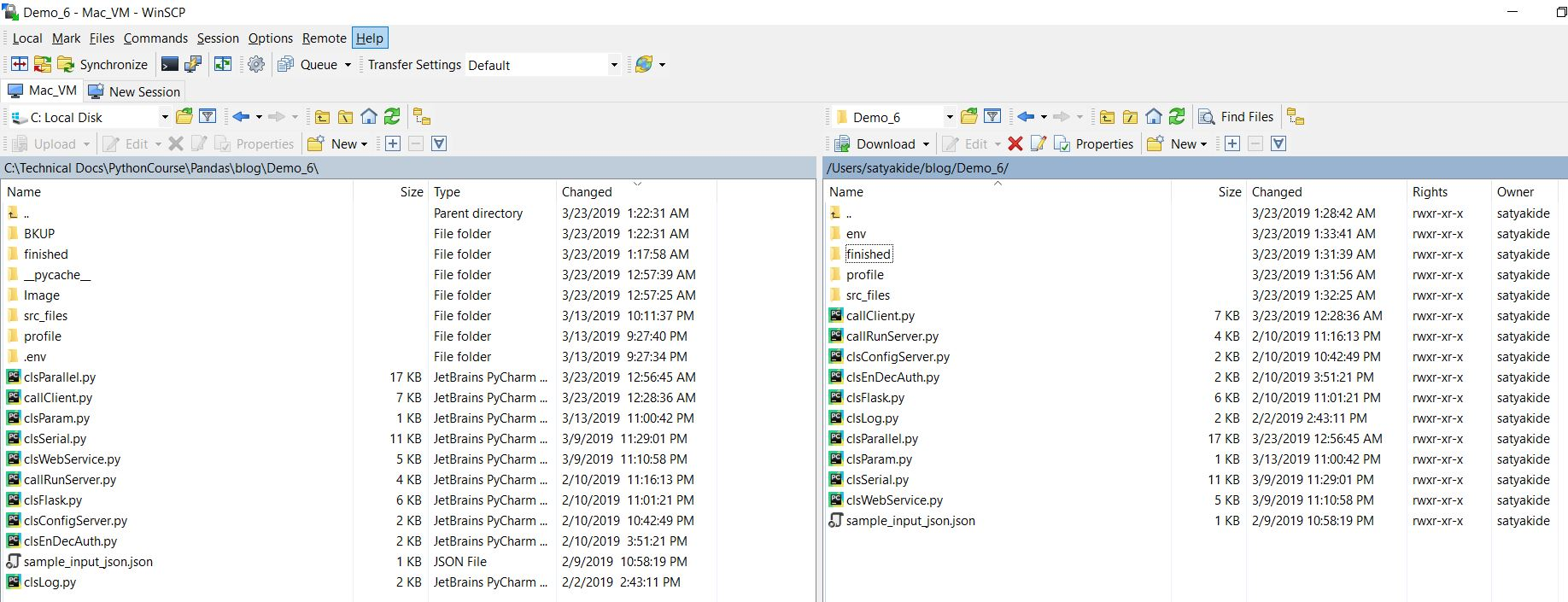##### Windows (16 GB – Core 2):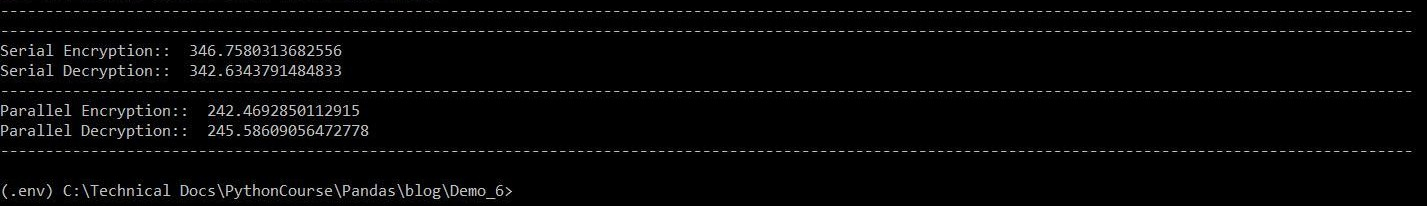##### Mac (10 GB – Core 2):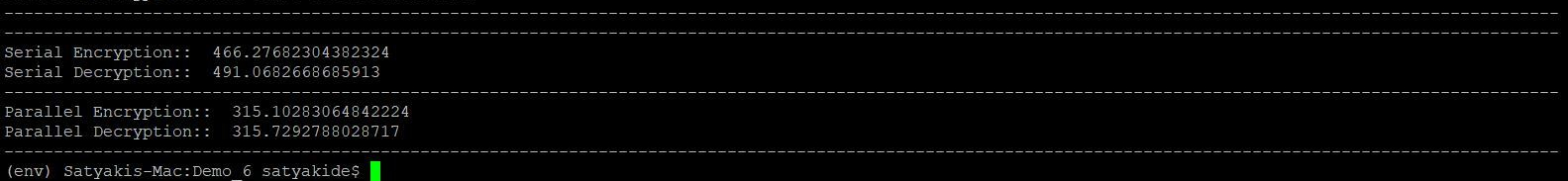##### Windows (16 GB – Core 2):##### Mac (10 GB – Core 2):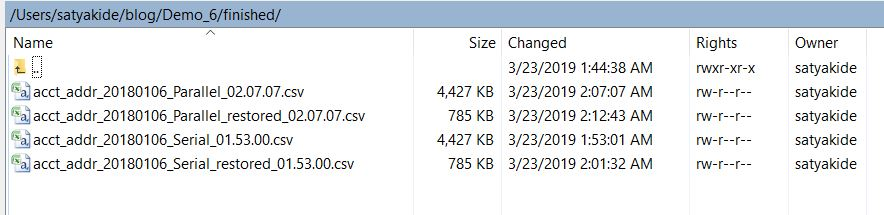Here is the final output –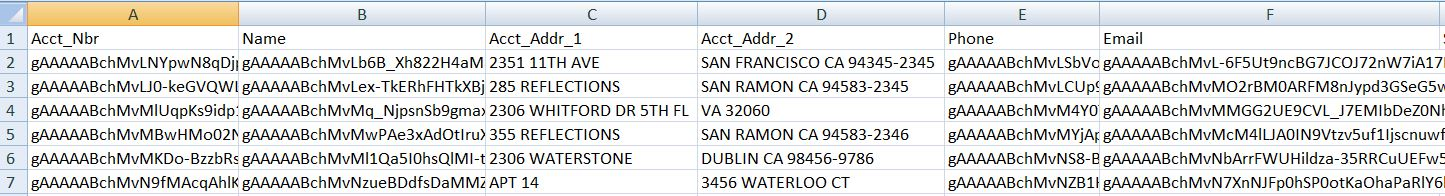So, we’ve achieved our target goal.